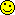<![CDATA[Math Is Fun Forum / Brute force for diophantine equation]]> 2023-02-05T09:34:08Z FluxBB https://www.mathisfunforum.com/viewtopic.php?id=25999 <![CDATA[Re: Brute force for diophantine equation]]> Yes why not if you would like to find out a solution to the following equation
2x³+6xy²-114=z³
Use Mathway to solve it
Thanks me later

]]>
https://www.mathisfunforum.com/profile.php?id=243804 2023-02-05T09:34:08Z https://www.mathisfunforum.com/viewtopic.php?pid=430686#p430686
<![CDATA[Re: Brute force for diophantine equation]]> This is an old thread, but maybe the methods in these posts that I found could help (basically transforming the equation into a elliptic curve, and using rational points on the curve to find other rational points which will eventually lead to a integral point).
https://mlzeng.com/an-interesting-equation.html
https://www.quora.com/How-do-you-find-t … Alon-Amit/

]]>
https://www.mathisfunforum.com/profile.php?id=222180 2022-04-11T01:55:58Z https://www.mathisfunforum.com/viewtopic.php?pid=424659#p424659
<![CDATA[Re: Brute force for diophantine equation]]> Thank you for your kind reply ?

]]>
https://www.mathisfunforum.com/profile.php?id=223593 2021-02-03T08:42:40Z https://www.mathisfunforum.com/viewtopic.php?pid=416752#p416752
<![CDATA[Re: Brute force for diophantine equation]]> sonu1997 wrote:

But can I send you a msg in your mail to discuss more about coding and brute force? If you don't mind

Sorry, sonu1997, but I'll decline your invitation.

I suggest you provide some more info for general discussion here, which could generate more interest in the thread and maybe even an outcome.

Btw, here's my code:

My clunky general code just uses Mathematica as the vehicle...

EDIT: Included a True/False check in my second code

]]>
https://www.mathisfunforum.com/profile.php?id=40741 2021-02-03T00:06:20Z https://www.mathisfunforum.com/viewtopic.php?pid=416745#p416745
<![CDATA[Re: Brute force for diophantine equation]]> Thanks a lot
But can I send you a msg in your mail to discuss more about coding and brute force? If you don't mind

]]>
https://www.mathisfunforum.com/profile.php?id=223593 2021-02-02T16:08:45Z https://www.mathisfunforum.com/viewtopic.php?pid=416738#p416738
<![CDATA[Re: Brute force for diophantine equation]]> Thanks, sonu1997, but I'll quit now.

I ran my brute force Mathematica code all last night and most of today for no results with x=0 to 1,000,000, y=0 to 1,000,000, c=114.

That was enough for me, and so I put the computer out of its misery and aborted the process midstream. I don't know how far into the computation I did that.

The whole exercise was taking up too much processing time with no reward to maintain my interest - sorry.

Btw, to find z, I was testing whether or not (2x³+6xy²-114)^(1/3) was an integer.

With my clunky nested 'For' loop code, that strategy worked quite well for smallish values of x and y, so I thought I'd try it on the above-mentioned larger x and y, not realising it would take forever.

I don't know Mathematica well enough to write efficient code, which it seems is needed to solve your problem in a reasonable time.

]]>
https://www.mathisfunforum.com/profile.php?id=40741 2021-02-02T12:09:25Z https://www.mathisfunforum.com/viewtopic.php?pid=416735#p416735
<![CDATA[Re: Brute force for diophantine equation]]> phrontister wrote:

Hi sonu1997 & irspow;

I also tried, and failed...but that doesn't mean much!I wonder if the equation's been entered correctly, though.

The reason I ask is that in case the trailing constant (which I'll call 'c') happens to be wrong, I tried a range of alternatives for c...with some success:

Using Mathematica, I found 31 solutions for {x,y,z}, subject to the following constraints:

1. x = between -100 & 100
2. y = between -100 & 100
3. c = between 100 and 999, containing at least two digits of the OP's '114' in their correct positions (in case c contains just one incorrect digit).

Three examples of my solutions:
(a) x = 7, y = ±48, z = 46, c = 118
(b) x = 10, y = ±69, z = 66, c = 164
(c) x = 5, y = ±4, z = 6, c = 514

Hlo phrontister,
Thanks for your efforts but let me tell you it is suspected that the magnitude of the solution is extremely large may be more than 15 digits so I'm sure you will never find any solution in between the range -100 ad 100 or even millions?
If you don't mind can I mail you?

]]>
https://www.mathisfunforum.com/profile.php?id=223593 2021-02-02T10:30:26Z https://www.mathisfunforum.com/viewtopic.php?pid=416730#p416730
<![CDATA[Re: Brute force for diophantine equation]]> irspow wrote:

Don’t feel bad. I was leery when I saw the trailing constant of 114 which has no rational cube root. Just for kicks I threw that equation into mathway and wolfram alpha, the two giants of online calculators. Neither of them could produce any rational solutions for z much less an integer.

No it's ok atleast you tried though. The solution happens to be very difficult

]]>
https://www.mathisfunforum.com/profile.php?id=223593 2021-02-02T10:27:03Z https://www.mathisfunforum.com/viewtopic.php?pid=416729#p416729
<![CDATA[Re: Brute force for diophantine equation]]> Hi sonu1997 & irspow;

I also tried, and failed...but that doesn't mean much!I wonder if the equation's been entered correctly, though.

The reason I ask is that in case the trailing constant (which I'll call 'c') happens to be wrong, I tried a range of alternatives for c...with some success:

Using Mathematica, I found 31 solutions for {x,y,z}, subject to the following constraints:

1. x = between -100 & 100
2. y = between -100 & 100
3. c = between 100 and 999, containing at least two digits of the OP's '114' in their correct positions (in case c contains just one incorrect digit).

Three examples of my solutions:
(a) x = 7, y = ±48, z = 46, c = 118
(b) x = 10, y = ±69, z = 66, c = 164
(c) x = 5, y = ±4, z = 6, c = 514

]]>
https://www.mathisfunforum.com/profile.php?id=40741 2021-02-02T03:46:04Z https://www.mathisfunforum.com/viewtopic.php?pid=416723#p416723
<![CDATA[Re: Brute force for diophantine equation]]> Don’t feel bad. I was leery when I saw the trailing constant of 114 which has no rational cube root. Just for kicks I threw that equation into mathway and wolfram alpha, the two giants of online calculators. Neither of them could produce any rational solutions for z much less an integer.

]]>
https://www.mathisfunforum.com/profile.php?id=2041 2021-01-31T21:53:37Z https://www.mathisfunforum.com/viewtopic.php?pid=416696#p416696
<![CDATA[Brute force for diophantine equation]]> Hey bro can you plz help me to find out a solution to the following equation
2x³+6xy²-114=z³
I tried many ways but failed only way left is using brute force.